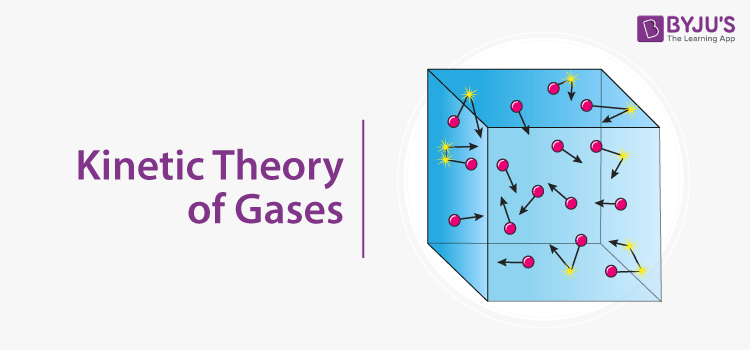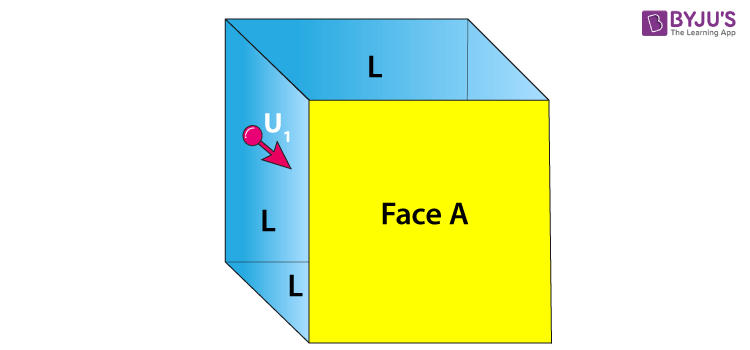# Kinetic Gas Equation Derivation## What Is Kinetic Theory Of Gases?

The kinetic theory of gases describes gas as a large number of submicroscopic particles such as atoms and molecules, all of which are in random and constant motion. The randomness arises from the particles collisions with each other and with the walls of the container.

## Derivation Of Kinetic Gas EquationConsider a cubical container of length ‘l ’ filled with gas molecules each having mass ‘m’ and let N be the total number of gas molecules in the container. Due to the influence of temperature, the gas molecules move in random directions with a velocity ‘v.’

The pressure of the gas molecules is the force exerted by the gas molecule per unit area of the wall of the container and is given by the equation

$P=\frac{F}{A}$

Let us consider a gas molecule moving in the x-direction towards face A. The molecule hits the wall with a velocity Vx and rebounds back with the same velocity Vx, and will experience a change of momentum which is equal to $\Delta p=-2mV_x$.

For a total of N number of gas molecules in the container, all such change in momentum is given by

$\Delta p=-2NmV_x$

The force is given by the equation

$F=\frac{\Delta p}{t}$

Therefore,

$F=\frac{-2NmV_{x}}{t}$

Gas molecules will hit the wall A and will travel back across the box, collide with the opposite face and hit face A again after a time t which is given by the equation

$t=\frac{2l}{V_x}$

Substituting the value of t in the force equation, we get the force on the molecules as

$F=\frac{-2NmV_x}{\frac{2l}{V_x}}$

$F_{molecules}=\frac{-2NmV_x}{\frac{2l}{V_x}}=\frac{-NmV_{x}^2}{l}$

Therefore, the force exerted on the wall is $F_{wall}=\frac{NmV_{x}^2}{l}$.

Now, the pressure P is given by the equation

$P=\frac{Force\,on\,the\,wall}{Area}=\frac{\frac{NmV_{x}^2}{l}}{l^2}=\frac{NmV_{x}^2}{l^3}$

Hence, $PV={NmV_{x}^2}$ (1)

Since Vx, Vy and VZ are independent speed in three directions and if we consider the gas molecules in bulk, then

$V_x^2=V_y^2=V_z^2$

Hence,

$V^2=3V_x^2$

Substituting the above condition in eq (1), we get

$PV=\frac{NmV^2}{3}$

Therefore,

$PV=\frac{1}{3}mNV^2$

This equation above is known as the kinetic theory equation.

The velocity V in the kinetic gas equation is known as the root-mean-square velocity and is given by the equation

$V_{rms}=\frac{\sqrt{V_1^2+V_2^2+V_3^2……..+V_n^2}}{N}$

We use this equation to calculate the root-mean-square velocity of gas molecules at any given temperature and pressure